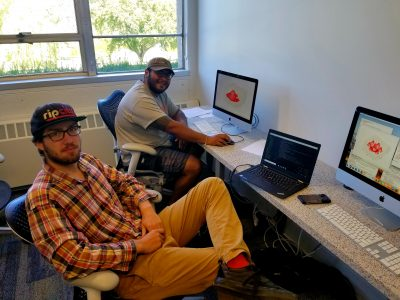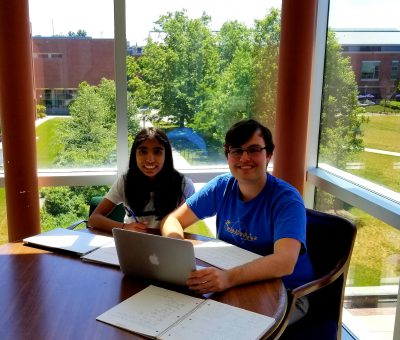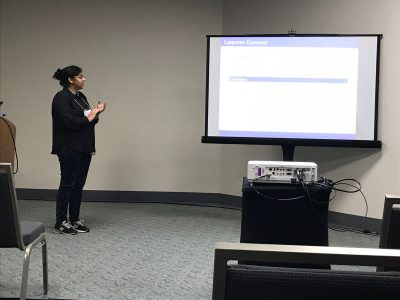# Geodesic interpolation on Sierpiński gaskets

• ### Laura A. LeGare

University of Notre Dame, USA

• ### Cory W. McCartan

Harvard University, Cambridge, USA

• ### Luke G. Rogers

University of Connecticut, Storrs, USA

• DOI 10.4171/JFG/100
• JFG VOL. 8, NO. 2 PP. 117–152
• 2018 project page

project page:

# Geodesic Interpolation on the Sierpinski Gasket

## Overview

Geodesics (shortest paths) on manifolds such as planes and spheres are well understood.  Geodesics on fractal sets such as the Sierpinski Triangle are much more complicated.  We begin by constructing algorithms for building shortest paths and provide explicit formulas for computing their lengths.  We then turn to the question of interpolation along geodesics—given two subsets of the Sierpinski Triangle, we “slide” points in one set along geodesics to the other set.  We construct a measure along the interpolated sets which formalizes a notion of the interpolation of a distribution of mass, and we prove interesting self-similarity relations about this measure.

# Computations on the Koch Snowflake with Boundary and Interior Energies

## Group Members## Overview

At the end of the 20th century studies had been conducted on the Koch Snowflake which had been motivated through work done by physicists on “fractal drum” experiments. These investigations focused on the eigenfunctions of the negative Dirichlet Lapcian generated on a planar domain with a fractal boundary, particularly with the condition that the boundary be set to zero. Here we study the eigenfunctions on the Koch Snowflake with a non-zero boundary condition and we consider a Laplacian defined on the boundary. To generate an n-level fractal and apply the Laplacian matrix, Python programming was implemented for both the n-level fractal and Laplacian matrix. This then gives us insight into the eigenvalues and visualization of the corresponding eigenfunctions on the Koch Snowflake. Initial observations indicate a kind of localization of the eigenfunctions on the fractal boundary.

# Lipschitz Continuity of Laplacian Eigenfunctions on a class of postcritically finite (PCF) self-similar sets

## Group Members## Overview

We prove a general result for Lipschitz and Hölder continuity of functions defined on a class of postcritically finite (PCF) self-similar sets. Intuition for this theorem comes from formulating arguments on the unit interval, which is well understood in a classical setting. We generalize these results for many kinds of self-similar sets endowed with different measures and metrics. As a corollary to this general result, we prove that eigenfunctions of the Laplacian on the harmonic Sierpinski Gasket are Lipschitz continuous.

# Probability, Analysis and Mathematical Physics on Fractals 2018

Each year we are looking for a group of undergraduate students to work on Probability, Analysis and Mathematical Physics on Fractals. The aim of the projects will be exploration of differential equations and various operators on fractal domains. Students in the project are supposed to have the usual background in linear algebra and differential equations. Knowledge of Matlab, Mathematica, other computer algebra systems, or programming, as well as proof writing, mathematical analysis, and probability may be helpful but is not required. Previous undergraduate work includes published papers on the eigenmodes (vibration modes) of the Laplacian (2nd derivative) of functions that live on Sierpinski gasket type fractals, and the electrical resistance of fractal networks, as well as work on Laplacians on projective limit spaces. The exact choice of the topics to study will depend on the students’ background and interests. Besides being interesting, taking part in a research project like this may be very useful in the future (for instance, when applying to graduate schools).

# Math UConn REU at JMM 2018

Two of our REU (2017 Stochastics) participants, Raji Majumdar and Anthony Sisti, will be presenting posters Applications of Multiplicative LLN and CLT for Random Matrices and Black Scholes using the Central Limit Theorem on Friday, January 12 at the MAA Student Poster Session, and both of them will be giving talks on Saturday, January 13 at the AMS Contributed Paper Session on Research in Applied Mathematics by Undergraduate and Post-Baccalaureate Students.

Their travel to the 2018 JMM has been made possible with the support of the MAA and UConn’s OUR travel grants.# Financial Math: Portfolio Optimization and Dynamic Programming

## Group Members

Ayelet Amiran, Skylyn Brock, Ryan Craver, Ugonna Ezeaka, Mary Wishart## Overview

Financial markets have asymmetry of information when it comes to the prices of assets. Some investors have more information about the future prices of assets at some terminal time. However, what is the value of this extra information?

We studied this anticipation in various models of markets in discrete time and found (with proof) the value of this information in general complete and incomplete markets. For special utility functions, which represent a person’s satisfaction, we calculated this information for both binomial (complete) and trinomial (incomplete) models.

## Publication

 Journal reference: Involve 12 (2019) 883-899 DOI: 10.2140/involve.2019.12.883

# arXiv:1808.03186

tba

## Poster

PosterFinMath2018